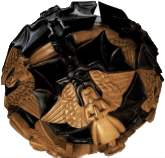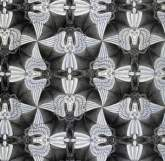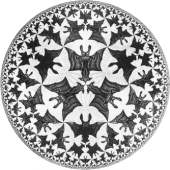# How Many Geometries Are There?

##### Age 16 to 18

Published 2001 Revised 2011

Just over 2000 years ago the Greek geometer Euclid laid down the foundations of geometry, and in doing so he made people aware of the idea that a mathematical statement needs to be proved. However convinced one might be about the truth of a statement, there is some possibility that one might be wrong, and so the only way to be certain is to give a proof.

Now if we are going to give proofs we must start somewhere; we cannot go on and on in a never ending attempt to justify what we are doing in terms of more and more basic mathematics. Euclid made the amazing step of realizing that mathematics needs axioms . An axiom is, roughly speaking, an agreed starting point which does not require proof; for example, we might agree that through any two points there is exactly one straight line, and that two lines meet in at most one point. In effect, what Euclid said was this: let us agree on some basic facts', and let us also agree that from then on everything else must be proved. With this in mind he then laid down the axioms of what we now call Euclidean geometry, and then went on to develop this geometry to a very high level indeed. This is the geometry that we learn at school, and which we use if, for example, we want to make a plan of a house.

All seems well, but suppose that we don't agree with Euclid's axioms; then what? Well, first, it doesn't invalidate Euclid's arguments for all he is claiming is that if we agree with his axioms , then such and such will follow. We cannot dispute that, even if we prefer our own axioms! It follows then that someone else could come along and change his axioms, and they would then presumably end up with a different theory. Let us try this out.

Suppose that we want to navigate round the earth, and that, being a navigator, we want to calculate distances, angles, and so on, on the surface of the earth. This means that we have to do some geometry on the surface of a sphere, and it is clear that Euclid's geometry will not work there. What we have to do, then, is to change the axioms and/or invent a new geometry. Let us invent a new geometry.

The path of shortest distance between two points is an arc of a great circle. On the surface of the earth the lines of longitude and the equator are great circles but other lines of latitude are not because they do not have their centres at the centre of the earth. In view of this it now seems reasonable to call the great circles the lines of our new spherical geometry . How many of these lines are there through the two points located at the north and south poles? There are infinitely many, of course, for every line of longitude gives us such a great circle, so here is one of Euclid's axioms that already we have had no option but to change!

Consider the triangle on the sphere with the vertices placed (1) at the north pole, (2) the point where the equator meets the Greenwich meridian, and (3) the point on the equator with longitude 90 degrees. The arcs of the three great circles that join these points should be considered as a spherical triangle for it is made up of three segments of the straight lines in our new geometry. However, each angle of the triangle is 90 degrees, so now we have a triangle with an angle sum of 270 degrees (and not 180 degrees)! Another of Euclid's results has gone! We can go on and on in this way; for example any two great circles (or lines in our geometry) meet in {two points} and again, this is not so in Euclid's geometry.

If you want to find out more about spherical geometry you might like to read the article 'When the angles of a triangle don't add up to 180 degrees.'

We have, I suggest, reached the point where we must agree that there are at least two different geometries, namely Euclidean geometry and spherical geometry. They do not contradict each other, and neither is right' or `wrong'; they simply represent a course of action advocated all those many years ago by Euclid, but with different starting points. What is surprising is that, considering the history of man's travels around the globe for so many years, it took us all so long after Euclid to realize that there are different, and equally valid, geometries.

So how should we answer the question in the title? Well, that is another, and very long, story but in the end there are very many geometries, each with its own peculiarities. Some are interesting and useful (for example, spherical geometry, and the curved space of Einstein's theory of relativity) and some are just curiosities. However, thanks to Euclid, who sadly never imagined such things, we know that they are all equally valid and each has a life of its own. I wonder where we would be now if the genius Euclid had been able to take this one extra step over 2000 years ago.

The artist M.C. Escher used the different geometries of the sphere the flat plane and the hyperbolic plane to give wonderful pictures. These are from The Magic of M.C. Escher, Thames and Hudson, 2000.Sphere with Angles and Devils (P.92)Regular Division of the Plane # 45 (P.93)Circle limit IV (Heaven and Hell) (P.180)

Circle limit IV (Heaven and Hell) (P.180) All M.C. Escher works (c) 2001 Cordon Art BV - Baarn - the Netherlands. All rights reserved. www.mcescher.com